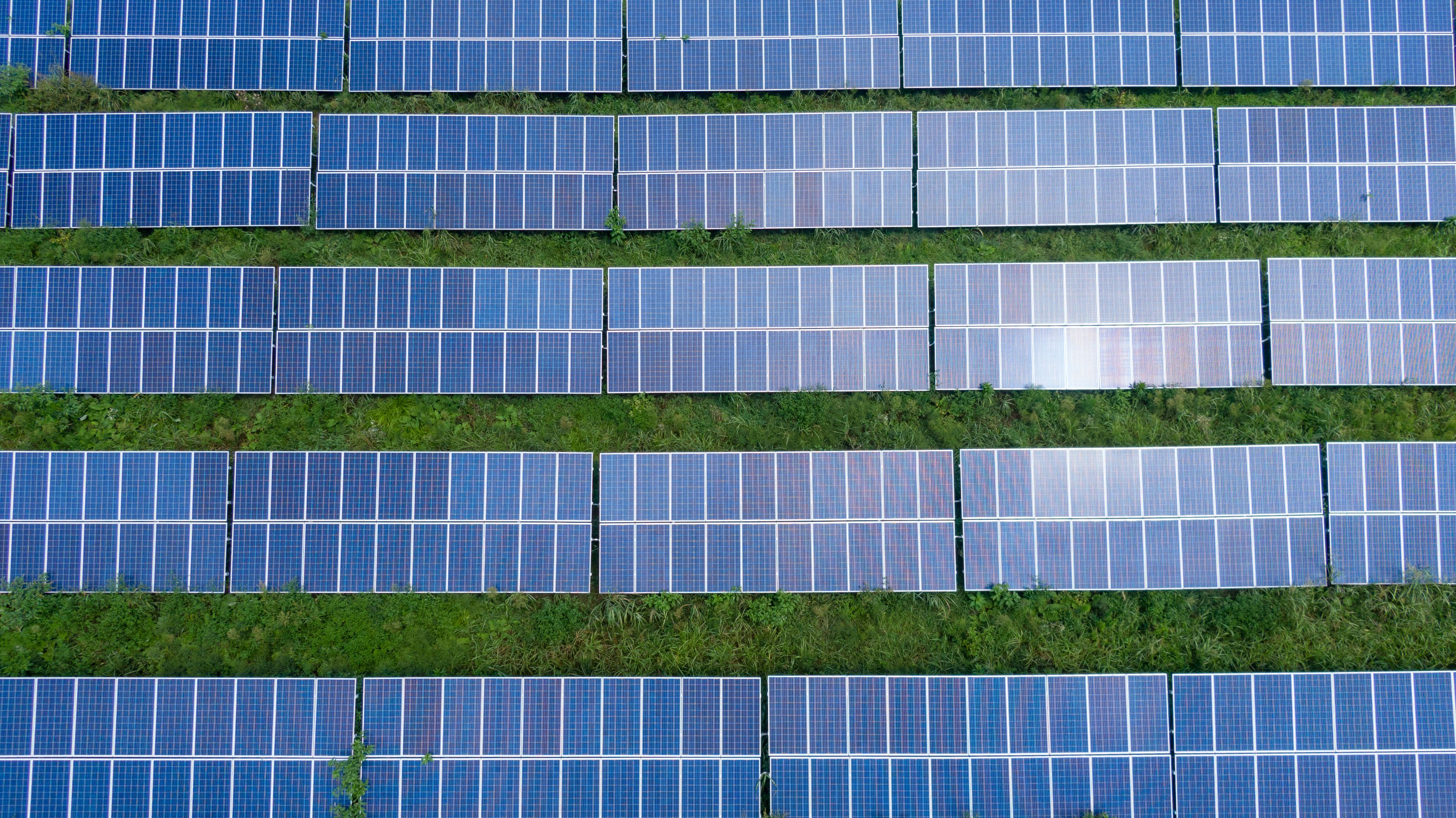## Mismatched Solar Panels- Same amps, different volts and watts

HI, this is Amy from the altE Store. Now we've
been doing a of videos where we are demonstrating how the best way is to wire
up mismatched . So we've already done you've got the same voltage but different
amps and watts, we've done the same watts, different amps and volts. Now we are going
to do the same amps, different volts and watts. Now definitely, with all of these tests that
we are doing, absolutely the best way to do this is to have each have its own independent
charge controller, if you've got very different solar panels. But, if that's not an option,
we're just giving you some ideas of how you can maximize it, and make the best out of
not the perfect situation. So we have two solar panels, a 100W 24V , with
a short circuit current of 3.03A, and an open circuit voltage of about 44V. And I've got
a 50W , with the Isc, the short circuit current also at 3.03A and it's Voc
is half of that, it's about 22V.

So, we are going to show you the power that they output
going through an MPPT charge controller, into my 12V battery. And then we will be able to
see independently how much power they put out and also how much power if
we wire then in and in parallel. Alright? Let's get started. Right now, I've got the
24V 100W solar connected through my MPPT charge controller to the battery, and I'm
reading 34.7V in, that makes sense, it's got a Vmp of 36V , and it's kind of hot out, so
the voltage is a little bit lower. And the current output is 5.86A. That's because it
took that higher voltage, and it dropped it down. So you've got your lower voltage coming
out, but your higher current coming out.

So that is 5.85A. So now let's change it around,
and connect the 12V 50W . OK, so now we've got our 12V 50W connected, and I'm
seeing 18V in, that makes perfect sense, it's got an 18Vmp. And the output is 3.08A. Now
it's got a 2.78A Imp, current at maximum power, so that means the MPPT charge controller is
actually boosting that current up a little bit, so we are getting better than standard
test conditions from this solar panel going through the MPPT charge controller. That's
kind of nice. So let's call it 3.08A on its own. Alright? And remember that we were up
almost at 6A with the 100W panel, makes sense, it's twice the watts, it was putting out twice
the current. So, let's wire the two of them together in parallel, and see what kind of
combined numbers we get coming out of it. Alright? <chatter> Alright, so we've got these two panels wired in parallel, the 24V 100W and the 12V50W, wired in parallel.Now you see the voltage going into the charge controller has actually been pulled way down. Remember
when we just had the 24V solar panel, we were up around 36V, 34V, somewhere around there.
And what are seeing now is 20V. So what's happening is this 12V solar panel, being wired
in parallel, is pulling that voltage way down. And because this is on the input of the charge
controller, unlike on the output of the charge controller where it drops the volts but raises
the amps, it's not increasing the amps. So we are just losing a tremendous amount of
power. Because watts equals volts times amps, if we are dropping the volts down, but leaving
the amps the same, we are losing a lot of power. So let's look at the output of this.
We are getting 6.29A coming out at 12.7V. That's, that's pretty lousy considering we
were getting with just the single panel in there.

So, let's wire the two of them in
now, going into that MPPT charge controller. Because they both have the same amps, but
different volts, we are going to keep the amps the same through the whole string, we're
going to add the volts together, and then the charge controller will drop those volts
down while increasing the amps. So let's see what we get with that, alright? The negative
from the 12V panel is going to the charge controller, the positive is going to the negative
of the 24V panel. And then the positive of that panel is going to the charge controller.
So you can see we've got 52V going into the charge controller, that's the addition of
the two panels wired together. And if we look at the current output, we're at 8.38A out
at 13V. So that's definitely the best power output that we've gotten. So wiring the two
solar panels, same amps, different volts, in series, into an MPPT charge controller
is the best solution if you can't give each of them their own charge controller. I hope
this was helpful. If so, give us a like and a share, and be sure to subscribe to our altE
Store channel so we will notify you when new videos are coming out.

And don't forget to
check us out at www.altestore.com, where we've been making renewable doable since 1999..